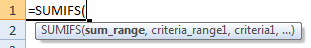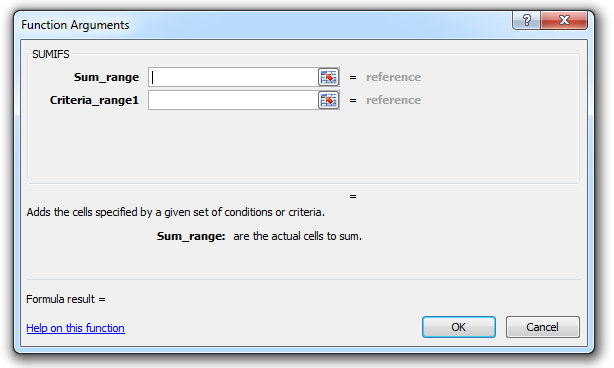### SUMIFS Function Examples in Excel, VBA, & Google Sheets

This Excel Tutorial demonstrates how to use the Excel SUMIFS Function in Excel to add numbers that meet multiple criteria, with formula examples.

## SUMIFS Function Description:

The SUMIFS Function Adds together all numbers that meet multiple criteria.

## Syntax and Arguments:

The Syntax for the SUMIFS Formula is:

`=SUMIFS(sum_range,criteria_range,criteria)`

### Function Arguments ( Inputs ):

sum_range – The range to sum.

criteria_range – The ranges containing the criteria that determines which numbers to sum.

criteria – The criteria indicating when to sum. Example: “<50" or "apples".

## SUMIFS Examples in VBA

You can also use the SUMIFS function in VBA. Type:
`application.worksheetfunction.sumifs(sum_range,criteria_range,criteria)`
For the function arguments (sum_range, etc.), you can either enter them directly into the function, or define variables to use instead.

## How to use the SUMIFS Function in Excel:

To use the AND Excel Worksheet Function, type the following into a cell:
`=AND(`
After entering it in the cell, notice how the AND formula inputs appear below the cell:You will need to enter these inputs into the function. The function inputs are covered in more detail in the next section. However, if you ever need more help with the function, after typing “=SUMIFS(” into a cell, without leaving the cell, use the shortcut CTRL + A (A for Arguments) to open the “Insert Function Dialog Box” for detailed instructions: